# How to use the LOG formula in Google SheetsNeeding to calculate the logarithm of your data inside spreadsheets? No need for a calculator; Google Sheets has its own LOG function to find the logarithm for you.

You may have already learned the behavior of natural logarithms and the usage of LN formula in Google Sheets. Although this formula is useful in many fields, it isn’t as flexible. With the natural logarithm, you cannot evaluate the logarithm for a base that is not Euler’s number (e).

What if we need a logarithm value for the base of 2? Or a base of 10? In such scenarios, the LOG formula in Google Sheets is the most flexible and useful function that can help us evaluate values for any base that we choose.

Without further ado, let’s explore exactly what the LOG function is in Google Sheets, when you can use it, and what it looks like when applied to your own Google Sheets data.

## What is the LOG function in Google Sheets?

The LOG function in Google Sheets returns the logarithm of a number in any base. However, in most cases, it’s usually used to calculate the logarithm of a number in base 10.

In the image below, you can see the difference between a natural logarithm (LN) and a logarithm (LOG), with two logarithms using a base of 2 and 10.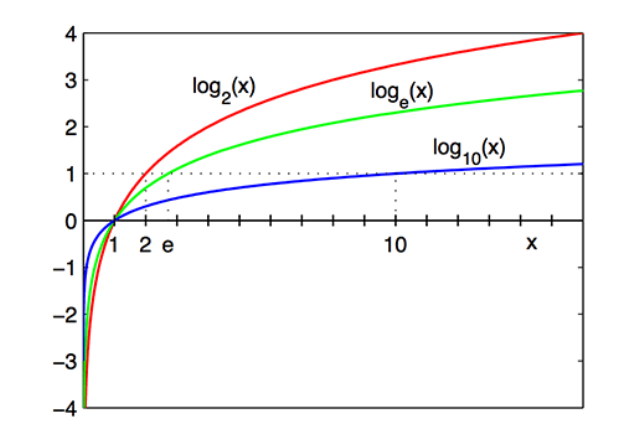Although complex in nature, the syntax of the LOG formula is simple.

### LOG function syntax

LOG(value, base)

• value – The value for which to calculate the logarithm given base.
• base The base to use for the calculation of the logarithm. Google Sheets uses the default base of 10.

It’s important to note that the value and base parameters inputs must be positive numeric values. The formula will return a #NUM! error if you try to use it with a zero or negative value and/or base.

### Usage: LOG formula in Google Sheets

Since this is a mathematical function, it gives us more perspective if we applied this practically on the Google Sheets application. So, here we go.

There are many different ways you can use the LOG function in Google Sheets. From a business perspective, you may use it as part of your calculation to return the percentage increase of a value over a given time. Alternatively, you could use the LOG function as part of a larger formula to calculate any value’s compound annual growth rate (CAGR).

### Use case of the LOG function in Google Sheets

Let’s take one of the methods above to show a real-life use case of when you can use the LOG function inside Google Sheets.

Let’s say I am the head of the finance department in my company. I want to calculate growth of the company’s latest investments as a percentage, to see whether the investments are worth it.

## How to use the LOG function in Google Sheets

Below is an example of how you can use the LOG function to return specific values. As you can see, I use the base of 10 across all the examples.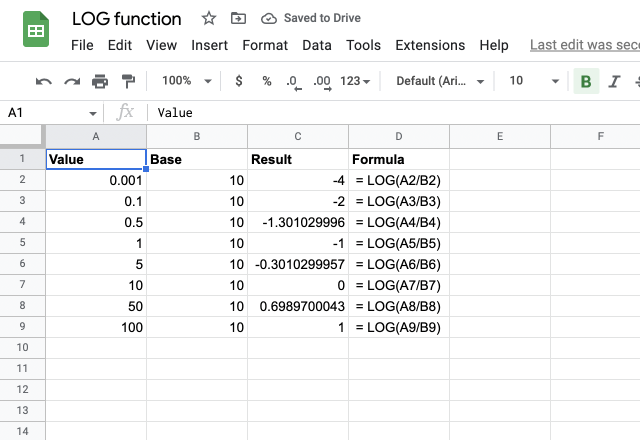As is the case with most Google Sheets formulas, the LOG formula also accepts direct numeric values, as well as cell references.

You may notice just by this data table that the results follow a similar exponential increase, as shown in the graph above.

If I plot my data as a line graph, you can see how it is almost a replica of the first graph.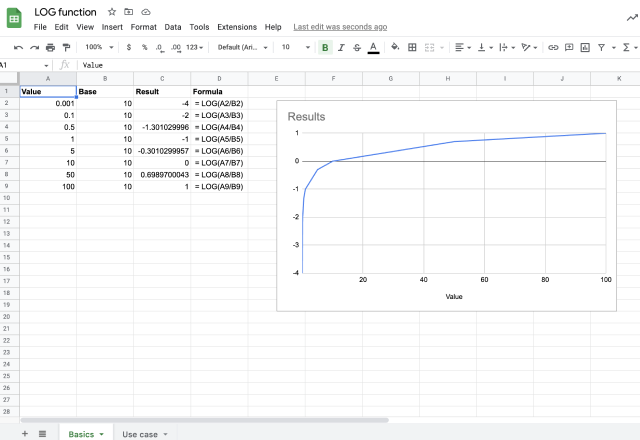## Examples of using the LOG function in Google Sheets

As the head of the finance department in my company, I want to calculate how long it would take for me to reach a certain amount of money.

### 1. How long would it take for you to double your investment?

In this example, I would like to know how long it would take for me to double my investment with a 1% monthly interest rate that compounds over time.

If I invested \$10,000, I would like to know how many months I would have to wait until I reach \$20,000. In this case, the principal sum would be \$10,000 and the final amount I want to reach would be \$20,000. The interest rate is 1%.

The result is the number of times interest is applied to the principal sum. In this case, it would take me over 69 months to double my investment at a 1% monthly interest rate.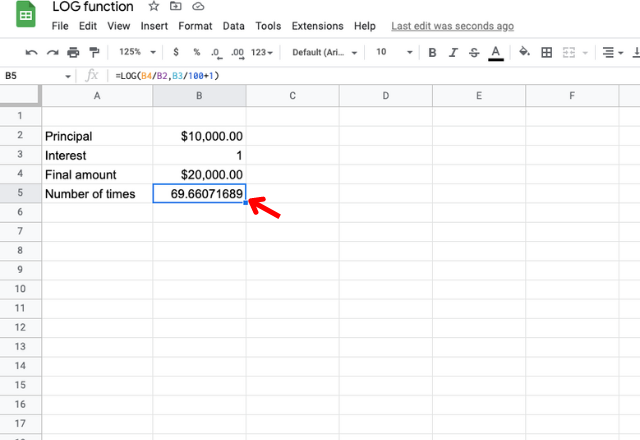We have applied the formula LOG(value, base). The value is the ratio between the final amount and the principal sum, whereas the base is the rate at which our capital will appreciate over time (the interest rate).

We have divided the final amount (B4) by the principal sum (cell B2) to get the correct value. In order to get the base, we have converted the interest rate to decimals (cell B3 divided by 100) and added 1.

As a result, we have used the formula as follows.

=LOG(B4/B2, B3/100+1)

### 2. How long would it take for you to turn \$10,000 into \$12,000?

Let’s say we want to turn \$10,000 into \$12,000. We are going to invest the principal sum in an investment that will yield a 2% monthly gain. In this case, it would take over 9 months to get the desired result.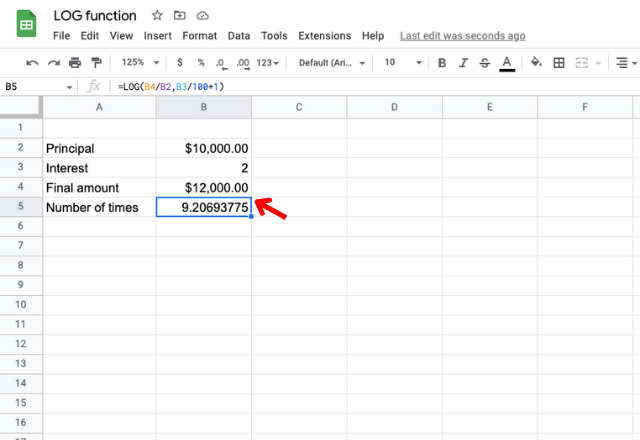## How to use the LOG formula in Google Sheets

There you have it! Now you can use the LOG formula in Google Sheets to perform some complex calculations.

If you want to calculate useful finance-related values quickly and easily, check out this article on how to use the FV formula in Google Sheets.